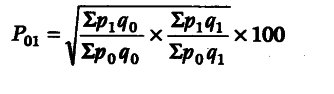# Discuss in brief, the methods of constructing weighted index number

Weighted Index Number These are the index number in which different items of the series are accorded different weight-age, depending upon their relative importance. Weighted index number certainly offer a much more realistic.
There are two methods of constructing weighted index number
(i) Weighted Average of Price Relative Method According to this method, weighted index number is simply the weighted arithmetic mean of price relative. In this method, weighted sum of the price relative is divided by the sum total of the weights.
Here, P_{ 01 } = ΣRW / ΣW
Here, P_{ 01 } = Index number for the current year in relation to the base year
W = Weight
R = Price relative .
(ii) Weighted Aggregative Method Under this method, weights are assigned to various items and instead of finding the simple aggregate of price, the weighted aggregate of the price are obtained. There are many methods to construct weighted index number such as Laspeyre’s method, Paasche’s method, Fisher’s method, Dorbish and Bowley’s method and Marshal and ’ Edgeworth’s method.

Here, we will discuss following methods
(a) Laspeyre’s Method This method involves the following steps
Step 1 Multiply the current year price of various commodities with base year weights and obtain Σ\$p_{ 1 }\$ q_{ 0 }
Step 2 Multiply base year price of various commodities with base year weights and obtain Σ\$p_{ 0 }\$ q_{ 0 }.
Step 3 Following formula is used to calculate index number
P_{ 01 } = Σ\$p_{ 1 }\$ q_{ 0 } / Σ\$p_{ 0 }\$ q_{ 0 } x 100
This will be the price index number.
(b) Paasche’s Method This method involves the following steps
Step 1 Multiply current year price of various commodities with current year weights and obtain Σ\$p_{ 1 }\$ q_{ 1 }
Step 2 Multiply the base year price of various commodities with the current year weights and obtain Σ\$p_{ 0 }\$ q_{ 1 }
Step 3 Following formula is used to calculate index number
P_{ 01 } = Σ\$p_{ 1 }\$ q_{ 1 } / Σ\$p_{ 0 }\$ q_{ 1 } X 100

© Fisher’s Method This method was introduced by Professor Irving Fisher. This method combines the techniques of Laspeyre’s method and Paasche’s method. Fisher used both base year as well as current year quantities as weight.
His formula to construct index number isyour ans is satisfactory but now my question is what is the importance of selection of weights in constructing aggregative p.i.n. formulae